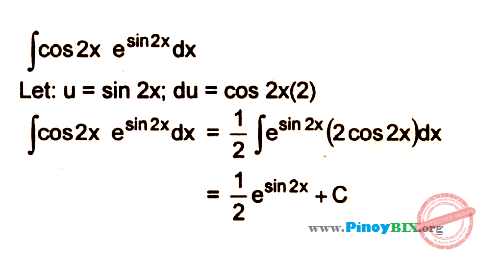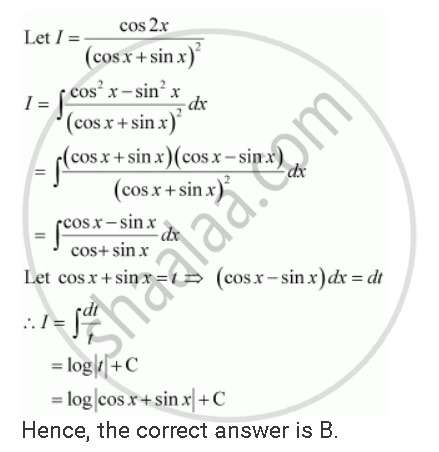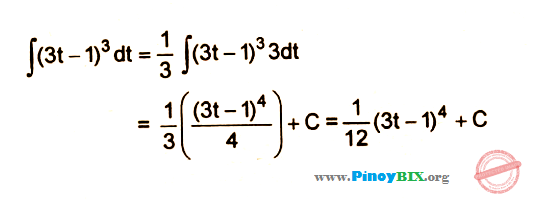Tuesday, 27 Sep 2022

# What is the integral of cos²x ?

One of the most dreaded mathematical concepts, integration is used to find the whole by uniting the parts. A lot of formulae and their application understanding the question make integration what it is. Although it is just the opposite of differentiation, the jump in difficulty is surprising. Finding the area bounded by graphs of functions also requires integration. So integration is a tough but an important idea in mathematics.

## What is the integral of cos²x?

We have to answer the question: what is the integral of cos²x? Follow the steps given below. First, write the integral of cos²x as ∫cos²x dx.

Then,

∫cos²x dx

= ½ ∫(2cos²x) dx

= ½ ∫(1 + cos2x) dx

= ∫(½ dx) + ½ ∫(cos2x dx)

= ½ x + ½ (sin2x)/2 + C

= x/2 + (sin2x)/4 + C

We write the function after an integral sign and before the suffix “dx”. Here, we write cos²x as ∫cos²x dx. Then we modify the function and bring it to a form which is solvable via integration formulae. cos²x becomes ½ (2cos²x) by multiplying and dividing the function by 2. Now, we modify this form to incorporate cos2x. This is because there is a way to integrate cos2x by using the formula meant for cosnx, where n stands for any number. Also, cos2x is equal to (2cos²x – 1), so, 2cos²x is equal to (1 + cos2x). The integral of this function is equal to the integral of 2cos²x.

(1 + cos2x) has two separate functions, and they can be operated separately because integration allows us to do so. Integral of 1 is simply x plus a constant, say, C1. For cos2x, we apply the formula for cosnx. Integral of cosnx is (sinnx)/n plus a constant. Differential of sinnx is ncosnx, so the opposite is integral of cosnx which is equal to (sinnx)/n plus a constant. Therefore, the integral of cos2x is (sin2x)/2 plus a constant, say, C2.

After the methods, we have the result as (x + (sin2x)/2 + C) (we combined C1 and C2). Finally, we multiply ½ with this result and get the integral of cos²x. So, the answer to our question that what is the integral of cos²x is

∫cos²x dx = (x/2 + (sin2x)/4 + C).

## What is the integral of cos²x by parts

The question that we have now is: what is the integral of cos²x by parts? To find the integral of cos²x by parts, follow the steps given below. First, write the integral of cos²x as ∫cos²x dx.

The steps are below:

Assume ∫cos²x dx equal to I.

Therefore,

I = ∫cos²x dx

= ∫(cosx × cosx) dx

= sinx cosx – ∫sinx (-sinx) dx

= ∫sin²x dx + sinx cosx

= sinx cosx + ∫1 dx – ∫cos²x dx

= sinx cosx + x + C – I

=> 2I = sinx cosx + x + C

=> I = (sinx cosx)/2 + x/2 + C

Thus, we can answer the question that what is the integral of cos²x by parts. The integral of cos²x by parts is

(sinx cosx)/2 + x/2 + C, where C is an arbitrary constant.

What happens here is that cos²x is written as the cosx multiplied by cosx. Then the two functions (cosx twice) are treated as being multiplied with each other and the method of integrating a function which has two functions being multiplied is applied. At last, one expression is brought in the right hand which equals the original function, and that is brought on the left hand side and added to the original expression. Then the left hand side coefficient is brought on the right hand side and we get the answer.

## What is the integral of 1/cos²x

The question now is not what is the integral of cos²x, but what is the integral of 1/cos²x. To find the integral of 1/cos²x, follow the steps below.

Write 1/cos²x as ∫(1/cos²x) dx.

∫(1/cos²x) dx.

= ∫sec²x dx.

= tanx + C

Finding out the integral of (1/cos²x) is very easy. However, the method is much different from what we do to know what is the integral of cos²x. The reciprocal of cosx is secx, and so that of cos²x is sec²x. Thus, we write (1/cos²x) as sec²x. The derivative of tanx is sec²x. Now, since integration is just the opposite of differentiation, we see the integral of sec²x is tanx. Then we attach a constant as usual. So, this is what happens here.

## What is the integral of cos2x

Follow the steps given below to find the integral of cos2x. But first, begin with writing cos2x as ∫cos2x dx.

∫cos2x dx

= (sin2x)/2 + C, where C is an arbitrary constant.

To find out the integral of cos2x, apply the integration formula ∫cosnx dx = (sinnx)/n. In our question, n is 2, and thus cosnx is co2x, and so on.

## What is the integral of cos2x e(sin2x) dxSolving this expression will require the method of substitution. Following this method, we substitute an expression within the given expression with some arbitrary letter, say, u. Then we differentiate u with respect to x and find du from there in terms of x, keeping it as close as possible to the rest of the expression after the expression we just substituted. After that, replace dx with the expression we just found. Then integrate this new expression and replace the u in the final answer with the original expression substituted with u.

For cos2x e(sin2x), first substitute sin2x with u, and then derive u with respect to x. In the process, find dx.

u = sin2x

=> du = 2cos2x dx

=> du/2 = cos2x dx

Now we replace cos2x dx with du/2, and sin2x with u, and get the expression

∫eu du/2 = ½ ∫eu du

Thus,

½ ∫eu du

= ½ eu + C

Now substituting u with sin2x, we get the integral of cos2x e(sin2x).

Therefore,

½ esin2x + C, where C is an arbitrary constant. And that is how we find the integral of cos2x e(sin2x).

## Integral of cos2x cos4x cos6x

Integrating cos2x cos4x cos6x takes quite a few mathematical manipulations to bring the expression to an integrable form. We resort to trigonometric formulae for our manipulations.

First, cos2x cos4x will be targeted. Trigonometric formula says

2cosA cosB = cos(A + B) + cos (A – B)

=> cosA cosB = (cos(A + B) + cos(A – B))/2.

So,

cos2x cos4x = (cos6x + cos(-2x))/2 = (cos6x + cos2x)/2.

Therefore,

cos2x cos4x cos6x = ((cos6x + cos2x)cos6x)/2 = (cos²6x + cos2x cos6x)/2.

Similarly,

cos2x cos6x = (cos8x + cos4x)/2,

and thus

(cos²6x + ½(cos8x + cos4x))/2.

Now convert cos²6x to an integrable form like cos8x and cos4x.

We know cos2nx = 2cos²nx – 1, and thus, cos²6x = (1 + cos12x)/2.

The function to integrate now is

(½(1 + cos12x) + ½ (cos8x + cos4x))/2

= ¼(1 + cos12x + cos8x + cos4x).

We can differentiate each of these functions independently using the formula ∫cosnx = (sinnx)/n.

Now, write the function as

∫¼(1 + (cos12x) + (cos8x) + (cos4x)) dx

= ¼(x + (sin12x)/12 + (sin8x)/8 + (sin4x)/4) + C

= x/4 + (sin12x)/48 + (sin8x)/32 + (sin4x)/16 + C, where C is an arbitrary constant.

We can integrate the function by targeting cos4x cos6x or cos2x cos6x also instead of cos2x cos4x in the beginning. The procedure will be the same as shown above. So, this is how we find the integral of cos2x cos4x cos6x.

## Integral of cos2x/(sinx+cosx)^2Solving this function to find its integral is easier than it seems. It only needs the knowledge of a basic trigonometric formula and a very handy integration formula. See the steps below.

Write cos2x/(sinx + cosx)² as ∫cos2x/(sinx + cosx)² dx first.

∫cos2x/(sinx + cosx)² dx

= (cos²x – sin²x)/(sinx + cosx)² dx

= (sinx + cosx)(cosx – sinx)/(sinx + cosx)² dx

= (cosx – sinx)/(sinx + cosx) dx

= log |sinx + cosx| + C.

What we do here is that we first convert cos2x to (cos²x – sin²x). Another algebraic manipulation gives us (cosx – sinx)(cosx + sinx) from (cos²x – sin²x). Then we cancel one (cosx + sinx) from above and the one of the same from the two below (the denominator has two (cosx + sinx)). The remainder is (cosx – sinx)/(cosx + sinx). Looking carefully, we will see the numerator is just the derivative of the denominator. For such an expression, there is an integration formula: ∫(f’(x)/f(x)) dx = log |f(x)| + C, where C is an arbitrary constant. The same is applied in the last while solving the given problem and thus the answer log |sinx + cosx| + C.

## What is the integral of cos2x sin2x dx

Solving this function to find out its integral again needs substitution. Follow the steps below to find out the integral of cos2x sin2x.

First write the function as ∫cos2x sin2x dx. Then substitute cos2x with u, and differentiate both sides, and find du in terms most close to the remaining function after cos2x.

u = cos2x

=> du = -2sin2x dx

=> – du/2 = sin2x dx

Now replaces cos2x with u, and sin2x dx with -du/2 in the function. The function becomes

-∫u/2 du. Now integrating this, we get:

-∫u/2 du = -u²/4 + C.

Now reinstate cos2x in place of u, and you will get the integral of cos2x sin2x.

Therefore, the integral is -cos²2x/4 + C, where C is an arbitrary constant.

## Integral of cos2x from 0 to pi/2

This question is a case of definite integration. We have a set of limits for this function. The answer will not have a constant at the end, and the integral value will in itself be a number. Let’s solve this using the steps below.

First write the function as ∫0π/2cos2x dx. The superscript after integration sign represents the upper limit, and the subscript after the same represents lower limit. Now integrate the function like an indefinite integration process, but write the limits outside the closing parenthesis as superscript and subscript after indefinite integration is done.

0π/2cos2x dx

= [(sin2x)/2]0π/2.

Now subtract the value of this function with the lower limit in place of x from the value of this function with the upper limit in place of x.

(sin2(π/2))/2 – (sin2(0))/2

= (sinπ)/2 – (sin0)/2

= (sinπ – sin0)/2

= (0 – 0)/2

= 0/2

= 0.

Our answer is 0. So the integral of cos2x from 0 to π/2 is 0.

## Integral of cos2x cos3x dxFinding the integral of cos2x cos3x needs the application of a common trigonometric formula:

2cosA cosB = cos(A + B) + cos(A – B)

=> cosA cosB = (cos(A + B) + cos(A – B))/2.

Let us start integrating cos2x cos3x using the steps below.

First write the function as

∫cos2x cos3x dx.

Then,

∫cos2x cos3x dx

= ½ ∫(cos5x + cosx) dx

= ½ ((sin5x)/5 + sinx) + C

= (sin5x)/10 + (sinx)/2 + C, where C is an arbitrary constant.

We first write the function with an integration sign as prefix and dx as suffix. Then use the trigonometric formula given above and convert the function to an integrable form. Then we evaluate the two separate expressions independently and find out the integral of cos2x cos3x. So the integral of cos2x cos3x is (sin5x)/10 + (sinx)/2 + C.

## What is the integral of (3t-1)^3 dtJust like every integration, we start by writing the function as ∫(3t-1)³ dt. The steps are as follows now:

This is a problem we can solve very easily using the method of substitution. First write (3t – 1) as u, and find dt in terms of du

(3t – 1) = u

=> 3dt = du

=> dt = du/3

Therefore,

∫(3t-1)³ dt

= ∫u³ du/3

= (u⁴/3)/4 + C

= u⁴/12 + C

= (3t – 1)⁴/12 + C

What we do here is we first do the integration by substitution bringing the function to an easily integrable form. Once the integration is done, we replace the substitute with the original function and get the final answer.

So, the integral of (3t – 1)³ is (3t – 1)⁴/12 + C, where C is an arbitrary constant.

## The integral of cos 4x cos xFor the solution of this function, we use a basic trigonometric formula which goes like:

2cosA cosB = cos(A + B) cos(A – B). Let us begin by writing the function to be integrated, or the integrand, as ∫cos4x cosx dx.

Thus,

∫cos4x cosx dx

= ½ ∫2cos4x cosx dx

= ∫(cos5x + cos3x)/2 dx

= ½ ∫cos5x dx + ½ ∫cos3x dx

= ½ (sin5x)/5 + ½ (sin3x)/3 + C

= (sin5x)/10 + (sin3x)/6 + C.

What we do here is a very simple trigonometric manipulation to bring the function to an integrable form. The formula when applied gives us the two cosine functions with an addition sign connecting them rather than their connection through their product. The new form is easy to integrate now because the two separate cosine functions can be integrated independent of each other. At the end, an arbitrary constant C is added.

Therefore, the integral of cos4x cosx is (sin5x)/10 + (sin3x)/6 + C.

## What is the integral of cos²x FAQs

### What is the integral of cos 2 theta?

Integral of cos2θ requires the following steps. First write the function you want to integrate as  ∫cos2θ dθ. Then use the standard integration formula for finding out the integral of cosnx.

∫cosnx dx = (sinnx)/n + C.

We apply the same formula to our function.

∫cos2θ dθ  = (sin2θ)/2 + C.

So the required integral is (sin2θ)/2 + C, where C is an arbitrary constant.

### What is the integration of Cos 3x?Integration of cos3x is very easy. Just follow the steps below:

First write the function as ∫cos3x dx.

Then,

∫cos3x dx = (sin3x)/3 + C.

The formula we use here is ∫cosnx dx = (sinnx)/n + C, where C is an arbitrary constant. So this is how we can calculate the integral of cos3x.

### What is the integral of sin2x?To find out the integral of sin2x, follow the steps below.

First write the function as ∫sin2x dx. Then use the integration formula

∫sinnx dx = -(cosnx)/n + C.

Therefore,

∫sin2x dx = -(cos2x)/2 + C.

So the answer is -(cos2x)/2 + C, where C is an arbitrary constant.

### What is the integration of 1?Integration of 1 is x + C, where C is an arbitrary constant.

### What is integral value?

Integral value is the value of a function by adding all its portions which were obtained by dividing the function into an infinite number of terms.

### Why is integral used?

The use of an integral is called integration. We use integrals in mathematics extensively to find quantities like area, volume, displacement, and so on. They are of two types: definite and indefinite. Indefinite integrals are used as anti-derivatives.